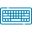# Double Integral CalculatorLimit for x

Limit for y

## Table of Content

Feedback

A double integral calculator calculates two dimensional integration with the two variables. Our double integral solver finds the area, volume, and average value of two variables over a given function.

## What is Double Integration?

“The method of integrating the volume of two dimensional spaces is referred to as a double integral”.

The functions of variables can be represented by ∫∫ and their functions over the two-dimensional space are f(x, y).

## Formula to Find the Double Integrals:

As we know there are definite and indefinite double integrals. In order to find the double definite integral the following formula is used and it is also written if you use the second variable first:

$$\int _{y_1}^{y_2}\left(\int _{x_1}^{x_2}f\left(x,y\right)dx\right)dy$$
$$\int _{x_1}^{x_2}\left(\int _{y_1}^{y_2}f\left(x,y\right)dy\right)dx$$

In the case of double indefinite integrals, the upper and lower functions can be used. So the equation is as follows:

$$\int\int f\left(x,y\right)dxdy$$

## Rule of Double Integration:

To integrate the double integral first integrate the innermost integral. For this purpose, there are a couple of methods to solving double integrals problems like integration by parts or integration by substitutions. So here is the simple rule that is considered by the double integral calculator with steps to evaluate the integration by parts is as follows:

$$∫∫m dn/dx dx. dy = ∫[mn -∫n dm/dx dx]dy$$

## Example:

$$\int\limits_{3}^{2}\int\limits_{2}^{1} \left(x^{3} + 5 x y^{2} + x y\right)\, dx\, dy$$

Indefinite Integral

$$=\frac{x^{2} y \left(3 x^{2} + 10 y^{2} + 3 y\right)}{12}+ \mathrm{constant}$$

Definite Integrals

$$=55$$

### Solution:

The double integral calculator considers the steps that are vital for integral:

First, we take the inner integral:

$$\int \left(x^{3} + 5 x y^{2} + x y\right)\, dx$$

Integrate term-by-term:

The integral of $$\frac{x^{n + 1}}{n + 1}$$ when $$n \neq -1$$

$$\int x^{3}\, dx = \frac{x^{4}}{4}$$

The double integral volume calculator evaluates the integral of constant times a function:

$$\int 5 x y^{2}\, dx = 5 y^{2} \int x\, dx$$

The integral of $$\frac{x^{n + 1}}{n + 1}$$ when $$n \neq -1$$

$$\int x\, dx = \frac{x^{2}}{2}$$

So, the result is:

$$\frac{5 x^{2} y^{2}}{2}$$

The integral of a constant times a function is the constant times the integral of the function:

$$\int x y\, dx = y \int x\, dx$$

The integral of $$x^{n}$$ is $$\frac{x^{n + 1}}{n + 1}$$ when $$n \neq -1$$

$$\int x\, dx = \frac{x^{2}}{2}$$

So, the result is:

$$\frac{x^{2} y}{2}$$

The result is: $$\frac{x^{4}}{4} + \frac{5 x^{2} y^{2}}{2} + \frac{x^{2} y}{2}$$

Now simplify:

$$\frac{x^{2} \left(x^{2} + 10 y^{2} + 2 y\right)}{4}$$

$$\frac{x^{2} \left(x^{2} + 10 y^{2} + 2 y\right)}{4}+ \mathrm{constant}$$

$$\frac{x^{2} \left(x^{2} + 10 y^{2} + 2 y\right)}{4}+ \mathrm{constant}$$

Steps for Second Integral:

$$\int \frac{x^{2} \left(x^{2} + 2 y \left(5 y + 1\right)\right)}{4}\, dy$$

The integral of a constant times a function is the constant times the integral of the function:

$$\int \frac{x^{2} \left(x^{2} + 2 y \left(5 y + 1\right)\right)}{4}\, dy = \frac{x^{2} \int \left(x^{2} + 2 y \left(5 y + 1\right)\right)\, dy}{4}$$

Integrate term-by-term:

The integral of a constant is the constant times the variable of integration:

$$\int x^{2}\, dy = x^{2} y$$

The integral of a constant times a function is the constant times the integral of the function:

$$\int 2 y \left(5 y + 1\right)\, dy = 2 \int y \left(5 y + 1\right)\, dy$$

Rewrite the integrand:

$$y \left(5 y + 1\right) = 5 y^{2} + y$$

Integrate term-by-term:

The integral of a constant times a function is the constant times the integral of the function:

$$\int 5 y^{2}\, dy = 5 \int y^{2}\, dy$$

The integral of y^n is $$\frac{y^{n + 1}}{n + 1}$$ when $$n \neq -1$$

$$\int y\, dy = \frac{y^{2}}{2}$$

The result is:

$$\frac{5 y^{3}}{3} + \frac{y^{2}}{2}$$

$$\frac{10 y^{3}}{3} + y^{2}$$

$$x^{2} y + \frac{10 y^{3}}{3} + y^{2}$$

$$\frac{x^{2} \left(x^{2} y + \frac{10 y^{3}}{3} + y^{2}\right)}{4}$$

Now simplify:

$$\frac{x^{2} y \left(3 x^{2} + 10 y^{2} + 3 y\right)}{12}$$

$$\frac{x^{2} y \left(3 x^{2} + 10 y^{2} + 3 y\right)}{12}+ \mathrm{constant}$$

$$\frac{x^{2} y \left(3 x^{2} + 10 y^{2} + 3 y\right)}{12}+ \mathrm{constant}$$

## Working of Double Integral Calculator:

It is extremely helpful to use the double integration calculator if you need fast results of analog for double integrals for two dimensions.

What to do:

• Chose the intervals either definite or indefinite
• Put the functions to integrate f(x, y)
• Set the limit values of certain functions
• Tap on “Calculate”

What you get:

• Definite and indefinite double integral
• Step-by-step calculations

## Attributes of Double Integration:

A double integral happens when the function with independent variables is integrated. This function is used by the second integral calculator to find the average value between two variables as well as with double integral area and volume. The properties of a double integral are as follows.

$$∫_ {x = a} ^b ∫_ {y = c} ^d f (x, y) dy . dx = ∫_ {y = c} ^d ∫_ {x = a} ^b f (x, y) dx . dy$$

$$∫∫( f(x, y) ± g (x, y)) dA = ∫∫ g (x,y) ± dA ∫∫ f(x, y) dA$$

If f(x, y) < g(x, y), then ∫∫g (x, y) dA > ∫∫ f(x, y) dA

$$k ∫∫ f (x, y) . dA = ∫∫ k. f(x ,y). dA$$

$$∫∫ R ∪ S f (x, y) . dA = ∫∫ R f (x, y). dA + ∫∫ sf (x, y). dA$$

## References:

Wikipedia: Double integrator, Differential equations, Transfer function representation, how to do double integrals.

Khan Academy: Double integrals, Volume under a surface, area under a curve, Two choices in direction, Sweeping area under a volume.### Sarah Taylor

I am a professional Chemist/Blogger & Content Writer. I love to research chemistry topics and help everyone learning Organic & Inorganic Chemistry and Biochemistry. I would do anything to spend vacations on a Hill Station.